# How to accelerate and compress neural networks with quantization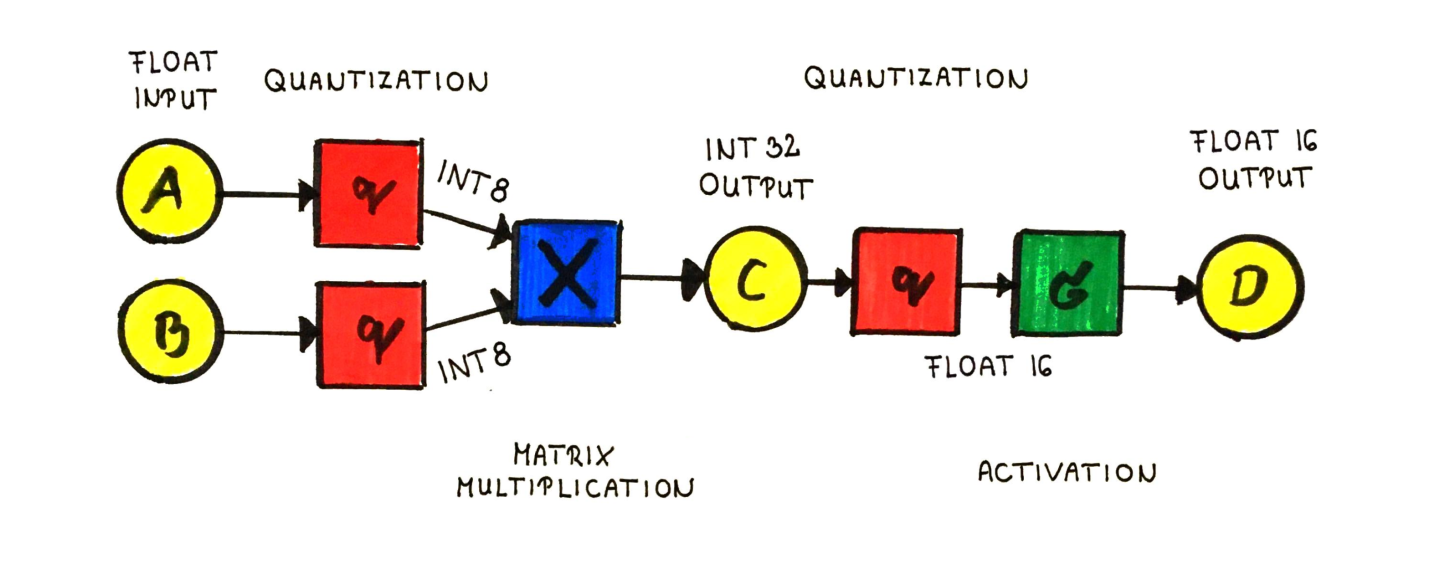Neural networks are very resource intensive algorithms. They not only incur significant computational costs, they also consume a lot of memory in addition.

Even though the commercially available computational resources increase day by day, optimizing the training and inference of deep neural networks is extremely important.

If we run our models in the cloud, we want to minimize the infrastructure costs and the carbon footprint. When we are running our models on the edge, network optimization becomes even more significant. If we have to run our models on smartphones or embedded devices, hardware limitations are immediately apparent.

Since more and more models move from the servers to the edge, reducing size and computational complexity is essential. One particular and fascinating technique is quantization, which replaces floating points with integers inside the network. In this post, we are going to see why they work and how can you do this in practice.

## Quantization

The fundamental idea behind quantization is that if we convert the weights and inputs into integer types, we consume less memory and on certain hardware, the calculations are faster.

However, there is a trade-off: with quantization, we can lose significant accuracy. We will dive into this later, but first let's see why quantization works.

### Integer vs floating point arithmetic

As you probably know, you can't just simply store numbers in the memory, only ones and zeros. So, to properly keep numbers and use them for computation, we must encode them.

There are two fundamental representations: integers and floating point numbers.

Integers are represented with their form in base-2 numeral system. Depending on the number of digits used, an integer can take up several different sizes. The most important are

• int8 or short (ranges from -128 to 127),
• uint8 (ranges from 0 to 255),
• int16 or long (ranges from -32768 to 32767),
• uint16 (ranges from 0 to 65535).

If we would like to represent real numbers, we have to give up perfect precision. To give an example, the number $1/3$ can be written in decimal form as $0.33333…$ with infinitely many digits, which cannot be represented in the memory. To handle this, floating-point numbers were introduced.

Essentially, a float is the scientific notation of the number in the form

$\text{significand} \times \text{base}^{\text{exponent}},$

where the base is most frequently $\textstyle 2$, but can be $\textstyle 10$ also. (For our purposes, it doesn't matter, but let's assume it is $\textstyle 2$.)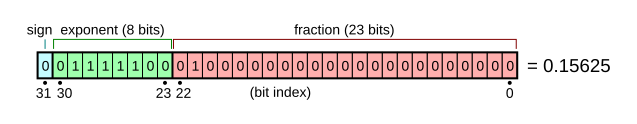Similarly to integers, there are different types of floats. The most commonly used are

• half or float16 (1 bit sign, 5 bit exponent, 10 bit significand, so 16 bits in total),
• single or float32 (1 bit sign, 8 bit exponent, 23 bit significand, so 32 bits in total),
• double or float64 (1 bit sign, 11 bit exponent, 52 bit significand, so 64 bits in total).

If you try to add and multiply two numbers together in the scientific format, you can see that float arithmetic is slightly more involved than integer arithmetic. In practice, the speed of each calculation very much depends on the actual hardware. For instance, a modern CPU in a desktop machine does float arithmetic as fast as integer arithmetic. On the other hand, GPUs are more optimized towards single precision float calculations. (Since this is the most prevalent type for computer graphics.)

Without being completely precise, it can be said that using int8 is typically faster than float32. However, float32 is used by default for training and inference for neural networks. (If you have trained a network before and didn't specify the types of parameters and inputs, it was most likely float32.) So, how can you convert a network from float32 to int8?

### Quantizing networks

The idea is very simple in principle. (Not so much in practice, as we'll see later.) Suppose that you have a layer with outputs in the range of $[-a, a)$, where a is any real number. First, we scale the output to $[-128, 128)$, then we simply round down. That is, we use the transformation

$x \mapsto \Big\lfloor 128 \frac{x}{a} \Big\rfloor.$

To give a concrete example, let's consider the calculation

$\begin{bmatrix} -0.18120981 & -0.29043840 \\ 0.49722983 & 0.22141714 \end{bmatrix} \begin{bmatrix} 0.77412377 \\ 0.49299395 \end{bmatrix} = \begin{bmatrix} -0.28346319 \\ 0.49407474 \end{bmatrix}.$

The range of the values here is $(-1, 1)$, so if we quantize the matrix and the input, we get

$\begin{bmatrix} -24 & -38 \\ 63 & 28 \end{bmatrix} \begin{bmatrix} 99 \\ 63 \end{bmatrix} = \begin{bmatrix} 4770 \\ 8001 \end{bmatrix}.$

This is where we see that the result is not an int8. Since multiplying two 8-bit integers is a 16-bit integer, we can de-quantize the result with the transformation

$x \mapsto \frac{ax}{16384}$

to obtain the result

$\begin{bmatrix} -0.2911377 \\ 0.48834229 \end{bmatrix}.$

As you can see, this is not exactly what we had originally. This is expected, as quantization is an approximation and we lose information in the process. However, this can be acceptable sometimes. Later, we will see how the model performance is impacted.

### Using different types for quantization

We have seen that quantization basically happens operation-wise. Going from float32 to int8 is not the only option, there are others, like from float32 to float16. These can be combined as well. For instance, you can quantize matrix multiplications to int8, while activations to float16.

Quantization is an approximation. In general, the closer the approximation, the less performance decay you can expect. If you quantize everything to float16, you cut the memory in half and probably you won't lose accuracy, but won't really gain speedup. On the other hand, quantizing with int8 can result in much faster inference, but the performance will probably be worse. In extreme scenarios, it won't even work and may require quantization-aware training.

## Quantization in practice

There are two principal ways to do quantization in practice.

1. Post-training: train the model using float32 weights and inputs, then quantize the weights. Its main advantage that it is simple to apply. Downside is, it can result in accuracy loss.
2. Quantization-aware training: quantize the weights during training. Here, even the gradients are calculated for the quantized weights. When applying int8 quantization, this has the best result, but it is more involved than the other option.

According to the TensorFlow Lite documentation, this is how these methods perform.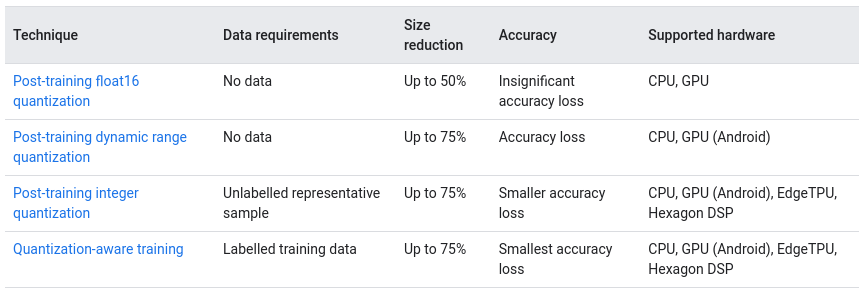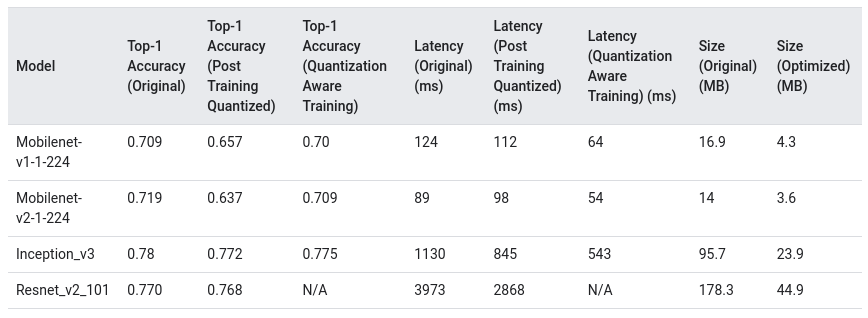In practice, the performance strongly depends on the hardware. A network quantized to int8 will perform much better on a processor specialized to integer calculations.

### Dangers of quantization

Although these techniques look very promising, one must take great care when applying them. Neural networks are extremely complicated functions, and even though they are continuous, they can change very rapidly. To illustrate this, let's revisit the legendary paper Visualizing the Loss Landscape of Neural Nets by Hao Li et al.

Below is their visualization of the loss landscape of a ResNet56 model without skip connections. The independent variables represent the weights of the model, while the the dependent variable is the loss.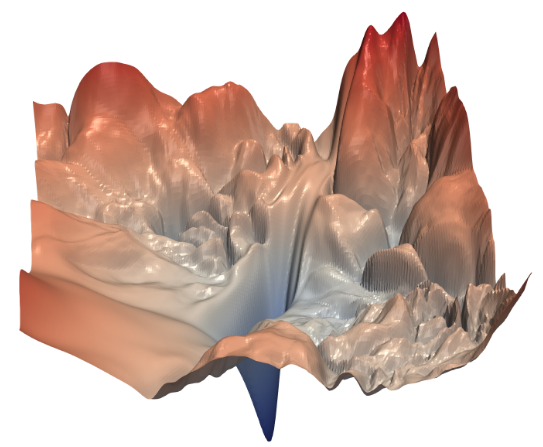This figure above illustrates the point perfectly. Even by changing the weights just a bit, the differences in loss can be enormous.

Upon quantization, this is exactly what we are doing: approximating the parameters by sacrificing precision for a compressed representation. There is no guarantee that it won't totally mess up the model in result.

As a consequence, if you are building deep networks for tasks where safety is critical and the loss of a wrong prediction is large, you have to be extremely careful.

### Quantization in modern deep learning frameworks

If you would like to experiment with these techniques, you don't have to implement things from scratch. One of the most established tools is the model optimization toolkit for TensorFlow Lite. This is packed with methods to squeeze down your models as small as possible. You can find its documentation here.

PyTorch also supports several quantization workflows. Although it is currently marked experimental, it is fully functional. (But expect the API to change until it is in the experimental state.) There is a great introductory article in the official PyTorch blog, you can find it here.

## Other optimization techniques

Aside from quantization, there are other techniques to compress your models and accelerate inference.

### Pruning

One particularly interesting one is weight pruning, where the connections of a network are iteratively removed during training. (Or post-training in some variations.) Surprisingly, you can remove even 99% of the weights in some cases and still have adequate performance.

### Knowledge distillation

The second major network-optimizing technique is knowledge distillation. Essentially, after the model is trained, a significantly smaller student model is trained to predict the original model.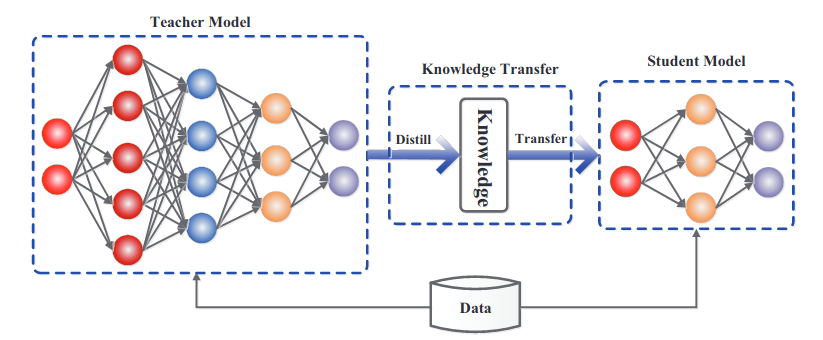Teacher and student models for knowledge distilling. Source: Knowledge Distillation: A Survey by Jianping Gou et al.

The knowledge distillation method was introduced by Geoffrey Hinton, Oriol Vinyals and Jeff Dean in their paper Distilling the Knowledge in a Neural Network.

Distilling has been successfully applied to compress BERT, a huge language representation model, which has applications all throughout the spectrum. With distilling, the model can actually be capable to be used on the edge, like smartphone devices.

One of the leaders in these efforts is the awesome Hugging Face, who are the authors of DistilBERT.

## Summary

As neural networks move from servers to the edge, optimizing speed and size is extremely important. Quantization is a technique which can achieve this. It replaces float32 parameters and inputs with other types, such as float16 or int8. With specialized hardware, inference can be made much faster compared to not quantized models.

However, since it quantization is an approximation, care must be taken. In certain situations, it can lead to significant accuracy loss.

Along with other model optimization methods such as weight pruning and knowledge distillation, this can be the quickest to use. With this tool under your belt, you can achieve results without retraining your model. In a scenario where post-training optimization is the only option, quantization can go a long way.

If you are interested in making neural networks fast by pruning weights or compressing, check out my following articles!